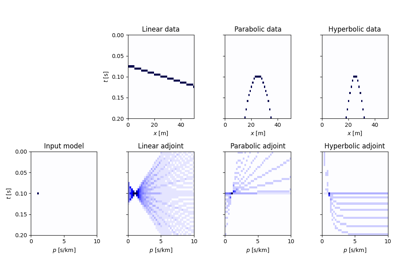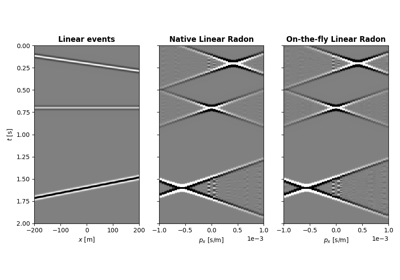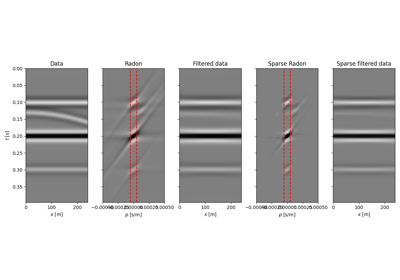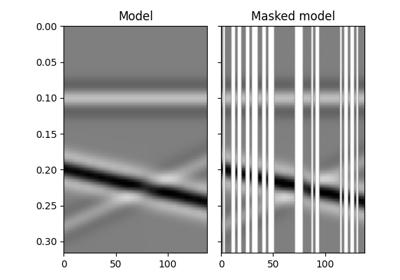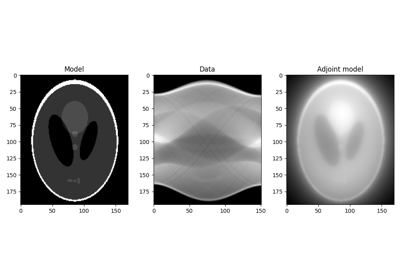class pylops.Spread(dims, dimsd, table=None, dtable=None, fh=None, interp=None, engine='numpy', dtype='float64', name='S')[source]#

Spread values from the input model vector arranged as a 2-dimensional array of size $$[n_{x_0} \times n_{t_0}]$$ into the data vector of size $$[n_x \times n_t]$$. Note that the value at each single pair $$(x_0, t_0)$$ in the input is spread over the entire $$x$$ axis in the output.

Spreading is performed along parametric curves provided as look-up table of pre-computed indices (table) or computed on-the-fly using a function handle (fh).

In adjont mode, values from the data vector are instead stacked along the same parametric curves.

Parameters
dimstuple

Dimensions of model vector (vector will be reshaped internally into a two-dimensional array of size $$[n_{x_0} \times n_{t_0}]$$, where the first dimension is the spreading direction)

dimsdtuple

Dimensions of data vector (vector will be reshaped internal into a two-dimensional array of size $$[n_x \times n_t]$$, where the first dimension is the stacking direction)

tablenp.ndarray, optional

Look-up table of indices of size $$[n_{x_0} \times n_{t_0} \times n_x]$$ (if None use function handle fh). When dtable is not provided, the data will be created as follows

data[ix, table[ix0, it0, ix]] += model[ix0, it0]


Note

When using table without dtable, its elements must be between 0 and $$n_{t_0} - 1$$ (or numpy.nan).

dtablenp.ndarray, optional

Look-up table of decimals remainders for linear interpolation of size $$[n_{x_0} \times n_{t_0} \times n_x]$$ (if None use function handle fh). When provided, the data will be created as follows

data[ix, table[ix0, it0, ix]]     += (1 - dtable[ix0, it0, ix]) * model[ix0, it0]
data[ix, table[ix0, it0, ix] + 1] +=      dtable[ix0, it0, ix]  * model[ix0, it0]


Note

When using table and dtable, the elements of table indices must be between 0 and $$n_{t_0} - 2$$ (or numpy.nan).

fhcallable, optional

If None will use look-up table table. When provided, should be a function which takes indices ix0 and it0 and returns an array of size $$n_x$$ containing each respective time index. Alternatively, if linear interpolation is required, it should output in addition to the time indices, a weight for interpolation with linear interpolation, to be used as follows

data[ix, index]     += (1 - dindices[ix]) * model[ix0, it0]
data[ix, index + 1] +=      dindices[ix]  * model[ix0, it0]


where index refers to a time index in the first array returned by fh and dindices refers to the weight in the second array returned by fh.

Note

When using fh with one output (time indices), the time indices must be between 0 and $$n_{t_0} - 1$$ (or numpy.nan). When using fh with two outputs (time indices and weights), they must be within the between 0 and $$n_{t_0} - 2$$ (or numpy.nan).

interpbool, optional

Use only if engine engine='numba'. Apply linear interpolation (True) or nearest interpolation (False) during stacking/spreading along parametric curve. When using engine="numpy", it will be inferred directly from fh or the presence of dtable.

enginestr, optional

Engine used for spread computation (numpy or numba). Note that numba can only be used when providing a look-up table

dtypestr, optional

Type of elements in input array.

namestr, optional

New in version 2.0.0.

Name of operator (to be used by pylops.utils.describe.describe)

Raises
KeyError

If engine is neither numpy nor numba

NotImplementedError

If both table and fh are not provided

ValueError

If table has shape different from $$[n_{x_0} \times n_{t_0} \times n_x]$$

Notes

The Spread operator applies the following linear transform in forward mode to the model vector after reshaping it into a 2-dimensional array of size $$[n_x \times n_t]$$:

$m(x_0, t_0) \rightarrow d(x, t=f(x_0, x, t_0)) \quad \forall x$

where $$f(x_0, x, t)$$ is a mapping function that returns a value $$t$$ given values $$x_0$$, $$x$$, and $$t_0$$. Note that for each $$(x_0, t_0)$$ pair, spreading is done over the entire $$x$$ axis in the data domain.

In adjoint mode, the model is reconstructed by means of the following stacking operation:

$m(x_0, t_0) = \int{d(x, t=f(x_0, x, t_0))} \,\mathrm{d}x$

Note that table (or fh) must return integer numbers representing indices in the axis $$t$$. However it also possible to perform linear interpolation as part of the spreading/stacking process by providing the decimal part of the mapping function ($$t - \lfloor t \rfloor$$) either in dtable input parameter or as second value in the return of fh function.

Attributes
shapetuple

Operator shape

explicitbool

Operator contains a matrix that can be solved explicitly (True) or not (False)

Methods

 __init__(dims, dimsd[, table, dtable, fh, ...]) adjoint() apply_columns(cols) Apply subset of columns of operator cond([uselobpcg]) Condition number of linear operator. conj() Complex conjugate operator div(y[, niter, densesolver]) Solve the linear problem $$\mathbf{y}=\mathbf{A}\mathbf{x}$$. dot(x) Matrix-matrix or matrix-vector multiplication. eigs([neigs, symmetric, niter, uselobpcg]) Most significant eigenvalues of linear operator. matmat(X) Matrix-matrix multiplication. matvec(x) Matrix-vector multiplication. reset_count() Reset counters rmatmat(X) Matrix-matrix multiplication. rmatvec(x) Adjoint matrix-vector multiplication. todense([backend]) Return dense matrix. toimag([forw, adj]) Imag operator toreal([forw, adj]) Real operator tosparse() Return sparse matrix. trace([neval, method, backend]) Trace of linear operator. transpose()

## Examples using pylops.Spread#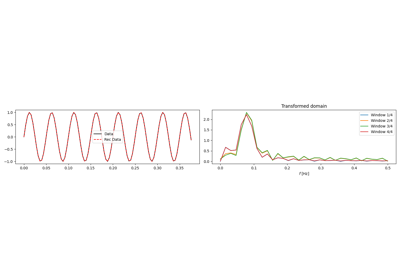1D, 2D and 3D Sliding

1D, 2D and 3D Sliding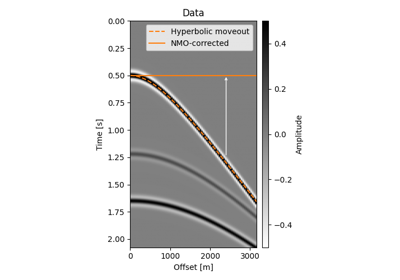Normal Moveout (NMO) Correction

Normal Moveout (NMO) Correction